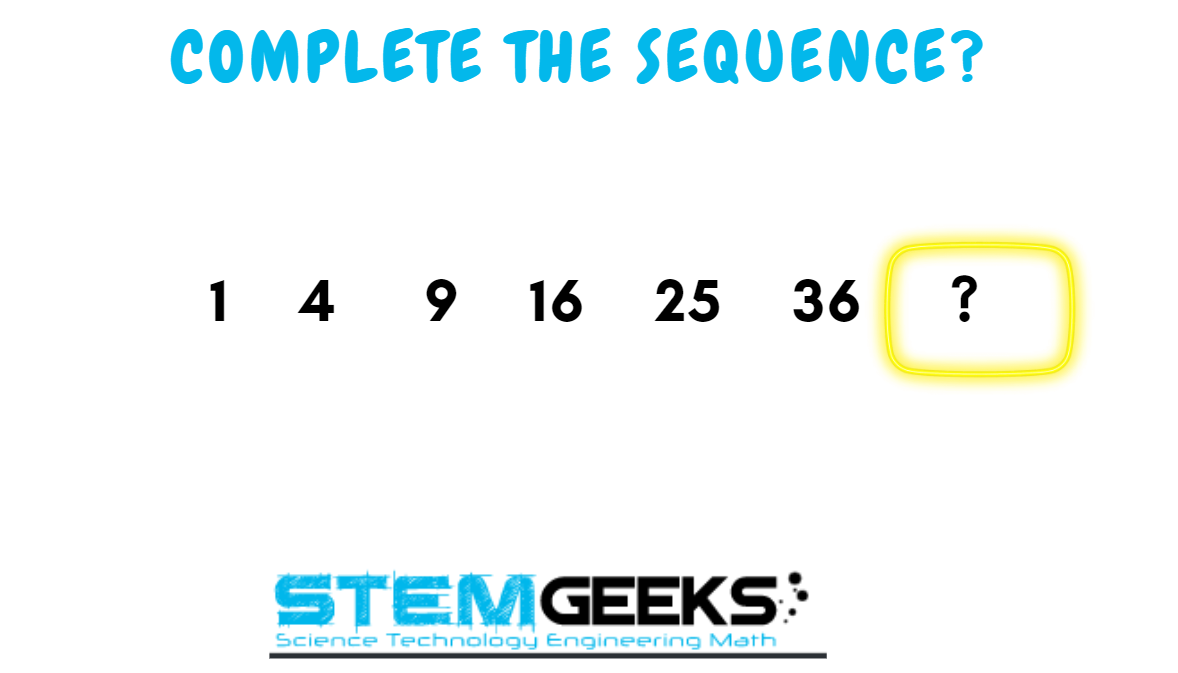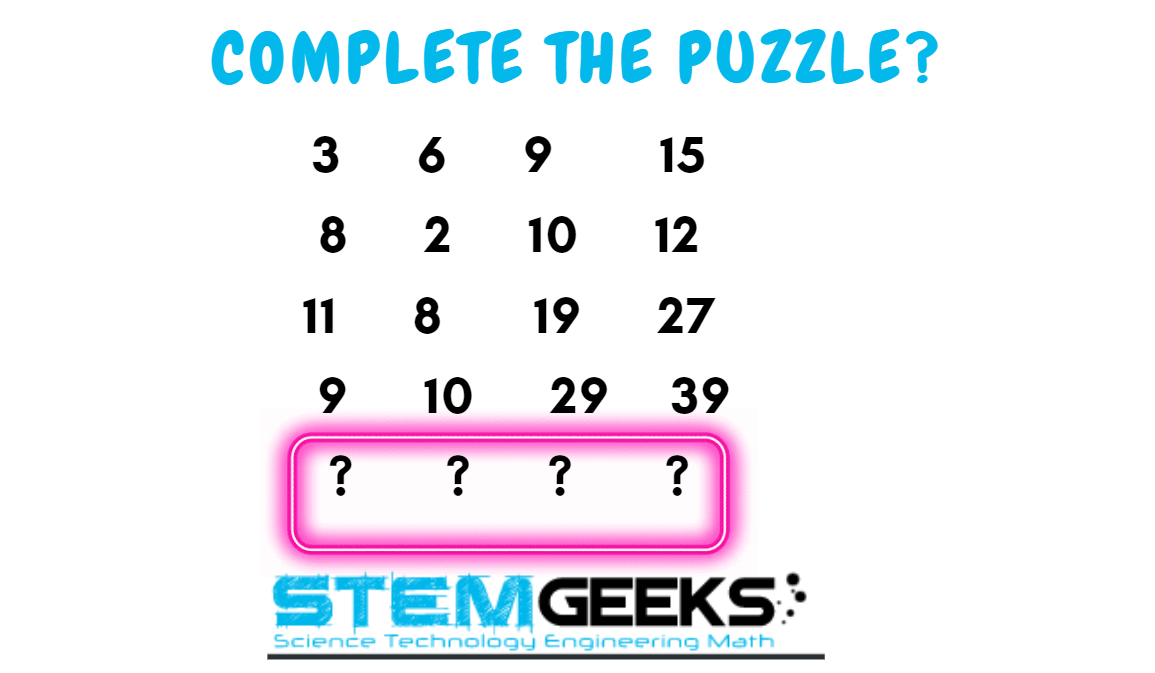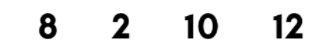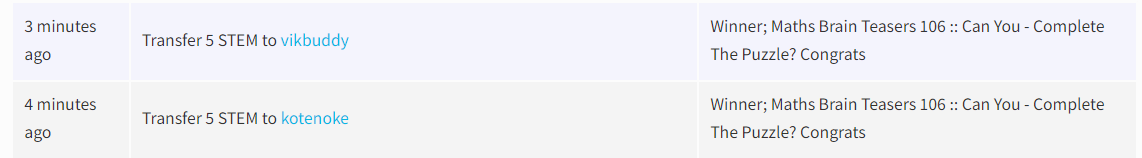Maths Brain Teasers 107 :: Can You - Decode the Logic to Complete the Sequence?Hey All; @StemGeeks Mathematician;

Without further, ado, let's get to our puzzle for today:: Maths Brain Teasers 107 :: Can You - Decode the Logic to Complete the Sequence?Have a close look at the image given and see if you can decode the logic given in the image to complete the puzzle. Try finding out the right NUMBER that Simplifies or Decodes the logic to the given puzzle. Replace the question marks with the right numbers so that it completes the series.

There is logic to resolve the puzzle; try finding out the logic and it should be resolved in seconds.. Guesswork isn't going to help...

With that, I'll leave you all with the Maths Brain Teasers 107 :: Can You - Decode the Logic to Complete the Sequence? Good luck solving the puzzle...

Maths Brain Teasers 106 :: Can You - Complete The Puzzle? - Solved with ExplanationLet's have a look at the Step by Step instructions to Solve the Maths Brain Teasers 106 :: Can You - Complete The Puzzle?

If you carefully look at the Image in question; You would have noticed the following pattern is being followed::

The logic was:: Starting from first row - add numbers corresponding to other number in the next row to get the next number

Lets' take an example of the first image::3 + 8 = 11 + 19 = 20

In a similar fashion we will work out for other rows::

15 + 12 = 27 + 39 = 66

Hence the answer to the Puzzle is Number:: 20 18 48 66

STEM token GiveAway

I'll be again doing a giveaway of STEM tokens to the lucky random winner with the correct answer. For the last contest, which was Maths Brain Teasers 106 :: Can You - Complete The Puzzle?.

We had only 4 entries and I am happy to see the engagement. At the same time, it's great to see the detailed answers provided by everyone as to how they arrived at the solution of the puzzle.. Well done guys.. Way to GO... Now the results part; TWO entries provided the right answer. And hence I'll split the reward between both the entries.Congratulations @vikbuddy && @kotenoke - You WIN yourself 5 STEM tokens. You should be having the rewards in your STEM Wallet Soon.

Math Quote for the Day::

Here is the motivation to solve the Maths Equation Puzzle?If you like my work, then please spread the Word.. that we do have the Math Brain Teasers competition here @StemGeeks platform. Reblog is much appreciated.

Best RegardsFind Me on the Other Social Media Platforms::PS:: All the Maths Brain Teasers; are made by me using the Pro Canva License Version

0
0
0.000(Edited)

49: Adding increasing odd numbers. Really loving these. Thanks so much for hosting. This old brain certainly needs the stimulation.

1+3=4
4+5=9
9+7=16
16+9=25
25+11=36
36+13=49

0
0
0.000It's increasing in odd numbers so the answer would be 49, I.e. 36 + 13

0
0
0.0000
0
0.000It's a Square series.
So ans is Square of 7 next, 49.

0
0
0.000(Edited)

Does it have something to do with a San Francisco based football team? Or maybe I am just too much of a square to figure this one out.

0
0
0.0001 (+3) 4 (+5) 9 (+7) 16 (+9) 25 (+11) 36 (+13) 49
it is a progression by addition of increasing odd numbers
!Gif odd

0
0
0.000Via Tenor

0
0
0.000